## 4680 Reputation

8 years, 131 days

## MaplePrimes Activity

### These are replies submitted by nm

You said

Eliminate hangs when using timelimit(). On long runs, random hangs happen when timelimit() do not expire as requested. Posted about this before.

I thought to post a typical example of this most annoying problem for me in Maple. Since it cause hangs in a script at places I have no control over. So when I run a script, I never know if it will hang or not and where as it seems to be random and it depends on the amount of timeout used.

I hope this gets fixed in next version of Maple. I have many many more such examples.

restart;
ode:=diff(y(t),t) = 1+y(t)+y(t)^2*cos(t);
sol:=dsolve(ode,y(t)):
timelimit(60,odetest(sol,ode));


And now it hangs. I waited for over 15 minutes, even though the time out is 60 seconds.

I know the solution is very complicated. But Maple should never hangs in a timelimit, in spite of what help says.

Note: In some cases, the execution may not abort at exactly the time limit imposed, but will abort as soon as it can do so safely.  This can happen when execution is in critical sections of certain built-in routines.

I am not asking for exact timeout ofcourse, but it should not be much more than 60 seconds, give and take few seconds.

I am sorry, but the above is just not acceptable for a software that has been around for more than 30 years now.

I worked on the development of the internals of a major operating system before. I know timeouts could always be implemented, no matter what the circumstances are, even if code is running inside a critical section.

In Mathematica for example, this never happens. https://reference.wolfram.com/language/ref/TimeConstraint.html and timeouts there always timeout. Each time and everytime.

Maple 2020.2 on windows 10.

Thank you

edit

After few more minutes, the above example did time out finally.  But took much much longer than the actual timelimit given. Waiting for say 20 minutes, when the timelimit is 1 minute is not right. As I said, it is not determistic. So each time it seems to behave differently.

thanks, I did not know about Latex:-Settings(leavespaceafterfunctionname = true).

I ended up actually using the above option after more testing. It turns out that \! does not work too well in mathjax.

I compile the latex to html using tex4ht, using mathjax mode. And notice the space between the function name and () is all gone now, in HTML. Not PDF. It seems this is not handed well by mathjax.

So I went back to using mleftright with the above option in place.

I think it might be a little more preferable to have the default Latex generated be as it was before, i.e. NO \! and have the user issue an explicit setting as above in order to add \! if they wanted to, instead of the other way around as it is now.

If you have TexLive installed and like to see this your self, here is a latex generated by Maple and the command to compile it to HTML under mathjax. This will work if you have TexLive 2020

\documentclass[12pt]{book}
\usepackage{amsmath}
\begin{document}

Let the constant of integration be called $c_2$ instead. Hence the above solution becomes
$w \! \left(x \right) = \frac{x}{3}+\frac{2}{3}+\frac{c_{2}}{\left(x +2\right)^{2}}$
Now, since $v^{\prime}\! \left(x \right) = w \! \left(x \right)$, then this
gives a new first order ODE to solve for $v \! \left(x \right)$
$v^{\prime}\! \left(x \right) = \frac{x}{3}+\frac{2}{3}+\frac{c_{2}}{\left(x +2\right)^{2}}$

\end{document}

THe command is

make4ht -ulm default -a debug foo3.tex "mathjax,htm"

This will create a file called foo3.htm in the same folder. If you view the HTML in browser, you'll see the problem.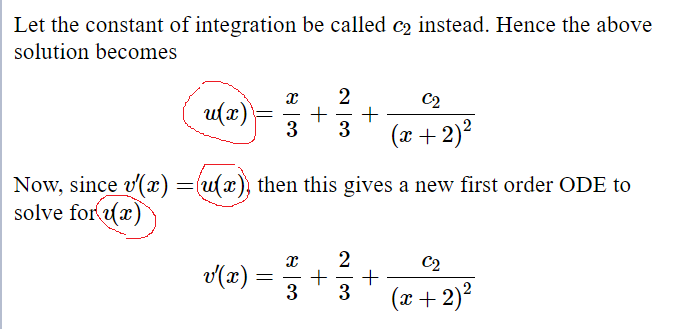Again, this might be a mathjax overcorrection for \! I do not know.  Removing \!  and compiling again, the problem is gone and the spacing is back to normal as it was before.

resolves issues with the installation of the Maplesoft Physics Updates

What was the cause of the issues?

I think that will be useful to know. Was it with windows itself?

great. thanks. I did not think of select at all. Much better than messing with op().  Now I can do

ode:=x^2*diff(y(x),x$2)-(x-3/16)*y(x)=0: ode:=expand(algsubs( y(x)=v(x)*x^(1/4)*exp(2*sqrt(x)),ode)); ode:=factor(ode); if type(lhs(ode),*) then ode_part :=select(has, lhs(ode), diff); rest_part :=remove(has, lhs(ode), diff) fi;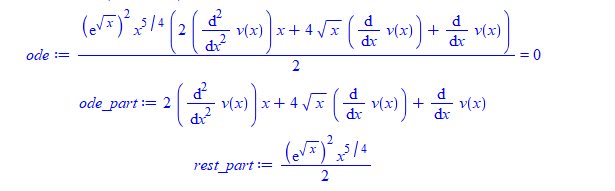## reply... it is a good idea to learn the Maple debugger. It is really very useful. There are commands there to stop at errors, and then you can examin variables and issue any Maple command while inside the debugger. Learning how to debug is part of learning programming. To step into the debugger, just so "stopat name_of_proc" and then call the proc. Now you are inside the debugger in new window. The help page gives all the commands that can be used in the debugger. f uses a 2nd argument, y, which is missing from the error message, it looks like you are not calling "f" correctly. ## reply... How do you actually use the code you posted? I am getting an error trying it foo:=proc( ic::(function(name)=algebraic) ) local y,x0,y0; y:=op(0,lhs(ic)); x0:=op(1,lhs(ic)); y0:=rhs(ic); print("y(x0) = y0 is", y(x0)=y0); end proc;  And now called it as foo( y(2)=0)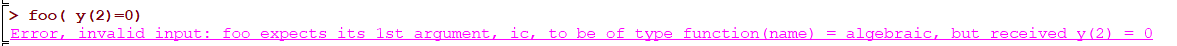I also tried it exactly as you have restart; IC:= (ic::(function(name)=algebraic)) -> op~([[1,0..1], 2], ic); ic:=y(0)=2; IC(ic)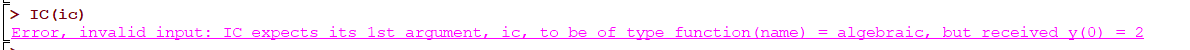I am using Maple 2020.1 on windows 10 ps. I am trying not to use patmatch as much, and each time I found a way not to use it, I do. ## reply... I am really confused now. I tried Latex:-Settings(powersoftrigonometricfunctions = computernotation); but it had no effect. Please see worksheet below. I thought also I am supposed to use textbooknotation in order to get cos(x)^3 to come out as \cos^{3}x. I must have been wrong. I am still trying to learn which option to use as this is all new. So if computernotation will do both, that is good. But why it is not working for me? I am using 870. I restarted Maple few times. No change. May be there is another setting that needs to be added somewhere to get same output as on you are getting?  > interface(version)> Physics:-Version();> #do I need to do something else other than this? Latex:-Settings(powersoftrigonometricfunctions = computernotation);> expr:=arccos(x); Physics:-Latex(expr)\cos^{-1}x  > expr:=cos(x)^3; Physics:-Latex(expr)\cos^{3}x  > Download latex_notation_871.mw ## reply... Thanks for the fix for the run-time error. Yes, It is fixed in 870. But now with 870, arccos() generates latex \cos^{-1} which is not a good notation at all and very confusing and no modern textbook uses this notation for inverse trig. This notation for inverse trig should not be even considered for any one of the options. The standard is to keep it \arccos as it was before, as in V 865 for example. When you say There is option 2: textbook notation only for trig (so cos(x)^2 is tex-translated as cos^2(x), but arccos(x) remains arccos, not cos^(-1)). Which is good and the ideal case. But this is not what I am seeing. I added this option, but it is still generating cos^{-1} for arccos? What Am I doing wrong? Why is the textbooknotation not doing what you are saying it is supposed to do and keep using arccos? Thank you  > interface(version)> Physics:-Version();> restart;  > Latex:-Settings()> Latex:-Settings(); Physics:-Latex:-UseImaginaryUnit:=i: Physics:-Latex:-UseColor := false: Latex:-Settings(powersoftrigonometricfunctions=textbooknotation): Latex:-Settings(usetypesettingcurrentsetting=true): Latex:-Settings();> expr:=sin(x)^3+arccos(1/x); Physics:-Latex(expr)\sin^{3}x +\cos^{-1}\left(\frac{1}{x}\right)  > expr:=sin(x)^3+arccos(x); Physics:-Latex(expr)\sin^{3}x +\cos^{-1}x  > ps. does one need to call Latex:-Settings(usetypesettingcurrentsetting=true): after changing a setting? I tried not calling it, and it made no difference. It is not clear what this option means exactly. So I tried both cases to find out. Download latex_notation_870.mw ## reply... Thanks for the fast improvement. I will test it tonight on a large PDF file build by generating Latex from Maple that I have of over 12,000 pages. I am sure it will be ok with the new version of Physics:-Latex. ## reply... I just checked one large latex file I have, and yes, it is only used for exp in this file. I thought I might have seen it somewhere else also sometime ago. May be with the old latex command? I am not sure now. But it is good if it is only used for exp, hopefully this will make it easier to modify in this case. As for \textrm vs. \mathrm, I would go with \mathrm based on reading the following references https://tex.stackexchange.com/questions/22350/difference-between-textrm-and-mathrm https://tex.stackexchange.com/questions/19502/is-there-a-preference-of-when-to-use-text-and-mathrm since e is a symbol meant to be in math mode, then mathrm seems the better choice. There are other subtle differences given in the above links. ## reply... I think you are doing it in no-optimal way. This is how I do these things. I generate all the plots, each plot in separate image file. I do all this in code. So I export each plot as eps file. (Maple still, in the year 2020, can't export, in code, a plot as PDF, only as eps file, which is really a very strange issue.). But one can export to eps and then convert to PDF after that using script. Once you are done generating the plots from Maple. Now close Maple. It is not needed any more. Next, I convert the eps files to pdf files, using script. Which there are number of ways to do. Next, I generate the table which contains the plots in Latex, using tabular or longtable and using includegraphics commands. Then compile the latex file to PDF. This will generate the table I want, as a PDF file. You get much much better quality table this way, with all the plots, much better sizing and control as you want. You can also add caption and get a professional looking output as you see in papers and books. Again, this is how I do this. The output is much better. All the above can be automated using a Makefile. You can see this post as an example of how to use tables of images in Latex https://tex.stackexchange.com/questions/64038/table-of-images-with-captions ## reply... So I suppose this does what you want for the ODE case? The use of Suppress is necessary for this to work - is that an issue? Great. Yes, that is exactly. I did not try Typesetting:-Suppress since I did not use it before. But it works. I could always later use Typesetting:-Unsuppress. restart; ic:={y(0)=0,D(y)(0)=1,(D@@2)(y)(0)=4}; Typesetting:-Settings(typesetprime=true); Typesetting:-Suppress(y(x)); Physics:-Latex(ic); ode:=diff(y(x),x)=sin(x); Typesetting:-Unsuppress(y(x)); Physics:-Latex(ode);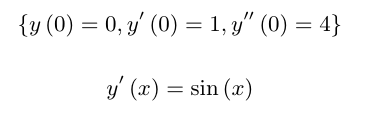This takes care of the ODE case. For PDE's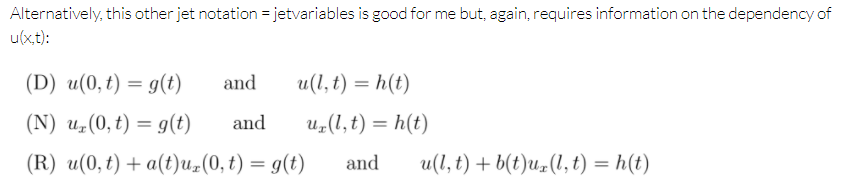Yes, I like this also and I used it also when writing latex by hand. But how do did you get Maple to generate the above Latex? Was the above generated by Maple? It is a good Latex. What is the exact code you used? I can't get the ToJet to accept the initial/boundary conditions in N and R you show above. I keep getting errors. About the ""not commas": in Jet notation. Yes, I see your point. May be a User option to suppress comma might be useful?. I only use single variable x,y,t,z etc.. for indepenent variables, that is why. But I uderstand your point. But for ODE's, issue is now closed. Thanks for help. ## reply... there is always the issue of who is the prime variable I think for ode's initial conditions, this is not needed to know? The input for ode IC is (using the D notation) is D(y)(x0)=y0 this can be translated to y'(x0)=y0 without the need to know the independent variable. Isn't this correct? When using eval() notation, no need to change that at all. For second order, the same, (D@@2)(y)(x0)=y0 can be translated to y''(x0)=y0 and so on. For non-integer order, such as nth order (D@@n)(y)(x0)=y0 is translated to y^(n)(x0)=y0 For PDE's, I did not know about ToJet. Thanks for the link. But that only works for the actual PDE, not for initial/boundary conditions. I could not make it translate PDE initial or boundary conditions. For example, pde := diff(u(x, y, t), t) = diff(u(x, y, t), x$2)+diff(u(x, y, t), y\$2) -  u(x,y,t);
Physics:-Latex(PDEtools:-ToJet(pde,u(x,y,t)));


works and gives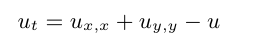(although a better notation is not to use the comma there and just have it as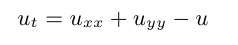But can't use ToJet for initial/boundary conditions

bc:=(D(u))(0, y, t) = 0;
Physics:-Latex(bc);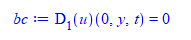But

PDEtools:-ToJet(bc,u(x,y,t));


gives error, since dependent variable now does not work

Error, (in PDEtools:-ToJet) found functions to be rewritten in jet notation, {u(0, y, t)}, having different dependency than the indicated in [u(x, y, t)]

And can not use PDEtools:-ToJet(bc,u(0,y,t)); it gives error.

The notation I like for initial/boundary conditions for PDE's are the standard ones as shown in Haberman PDE's books. Here is an example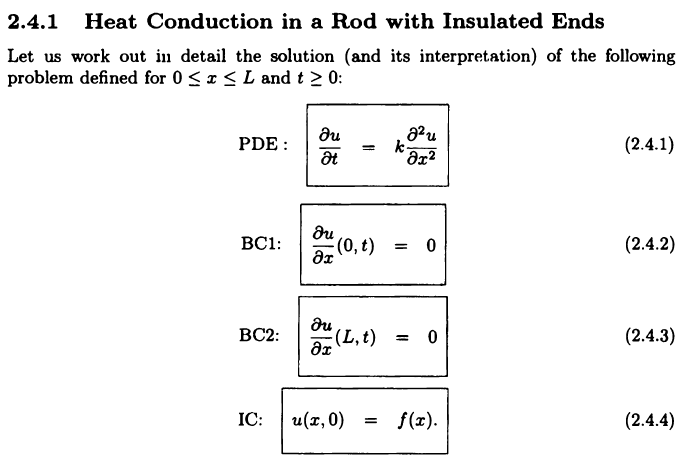But for non-initial conditions, for pde's, I like ToJet notations. (Just wish there was no comma in there)

Thank you

Yes, manually trying to convert it using string tools is always a possibility.

But need to be carefull to make sure it covers all cases and forms possible.

For example, your method only works for first order. Not higher.

restart;
ic:=[y(0)=0,D(y)(0)=1,(D@@2)(y)(0)=3];
s:=Physics:-Latex(ic, output=string);
s1:=StringTools:-SubstituteAll(s, "D\\left(y \\right)", "y'");



gives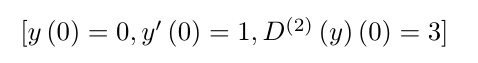But I am sure with more string manipulation this could be overcome. (need to figure the derivative order, and add as many '' as needed and so on.

Or I could simply add more cases. Like this

restart;
ic:=[y(0)=0,D(y)(0)=1,(D@@2)(y)(0)=3];
s:=Physics:-Latex(ic, output=string);
s1:=StringTools:-SubstituteAll(s, "D\\left(y \\right)", "y'");
s1:=StringTools:-SubstituteAll(s1, "D^{\\left(2\\right)}\\left(y \\right)", "y''");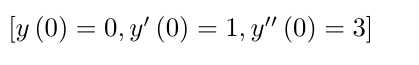I do not expect to have more than third order initial conditions. So this could work. I could add 2 additional string replacements.

I was hoping to avoid doing it manually by hand. But may be for now, this might be only option to get better Latex for this. I need to test this more in my code to see if it covers all the problems I have, as I have thousands of them.

However, the computer still handles (sin@@2)(x) and also the (operator form) "sin^2 * x" and we need a representation for those too.

This is the first time I see that one can write sin^2 * x in Maple as input. I have no idea what this represents or mean. I also did not know one can do (sin@@2)(x)  and that seems to have different semantics from sin(x)^2. I have no idea why one would actually use these in Maple for as I never used them.

In this case, I do not know what to suggest.

I was simply talking about the single argument trig/hyper trig functions, which are the familar ones such as sin(x)^n.

If the Latex conversion can somehow internally distiguish these functions only from the other forms you show and convert only these to \sin^n x, then will cover the majority of cases in normal practical usage of Maple, I would think, while leaving everything else the same as it is now.

If this is not possible, then I do not know what to suggest,.

I tried these two cases in Mathematica: sin(x)^3 (standard) vs. sin^3*x  to see how Mathematica Latex output is different: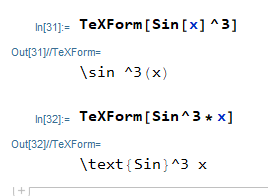So in Mathematica, it knew that the first case is the standard usage and used \sin for the output, but for sin^3*x (which you call operator form), it used \text{Sin} there. and those will typeset slightly different in output.

I did not know how to translate your other example of sin(@@3)(x) to Mathematica to try it.

Btw, having the latex for sin(x)^3 be  \sin^3 x  vs. \sin^3 (x)  is not as important as the location of exponent. I myself perefer the first one above. But the second one is also fine if it makes the conversion easier.

Only when the argument contains 2 or more symbols, then adding an explicit () is better. So sin(x+y)^3 translate to \sin^3(x+y) instead of \sin^3 x+y to remove ambiguity.

But any way, thanks for considering this and looking at it. I was only suggesting something to help make the latex better looking and I did not know about these other forms.

I made this table FYI. Only suggestion I could offer now, is to use \texttt for those functions when in operator form while using standard \sin or \cos, etc.. for the normal usages. This will make the latex output a little different. Not sure what else to think of at this time.